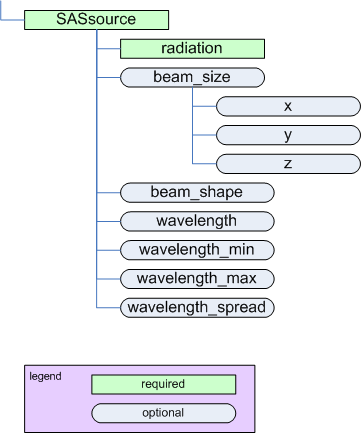SASinstrument

SAScollimation

# SASsource¶

parent:
SASinstrumentThe SASsource element

Name Type Occurrence Description Attributes

Name of the radiation used. For maximum compatibility with NeXus, use one of the types defined by NeXus NXsource: 

• Spallation Neutron Source
• Pulsed Reactor Neutron Source
• Reactor Neutron Source
• Synchrotron X-ray Source
• Pulsed Muon Source
• Rotating Anode X-ray
• Fixed Tube X-ray

or the NeXus probe type:

• neutron
• x-ray
• muon
• electron

beam_size container [0..1] Physical dimension of the beam (incident on the sample). If beam is round, just use X dimension. name={name} 
beam_shape string [0..1] Text description of the shape of the beam (incident on the sample).
wavelength float [0..1] wavelength ($$\lambda$$) of radiation incident on the sample. unit={unit} 
wavelength_min float [0..1] Some facilities specify wavelength using a range. The minimum of such a range is given by* wavelength_min*. unit={unit} 
wavelength_max float [0..1] Some facilities specify wavelength using a range. The maximum of such a range is given by* wavelength_max*. unit={unit} 
wavelength_spread float [0..1] Some facilities specify the width of the wavelength spectrum. The width of such a range is given by wavelength_spread. unit={unit} 

## geometry¶

See the figures in Definition of the coordinate axes.

## beam_size¶

Name Type Occurrence Description Attributes
$$x$$ float [0..1] Dimension of the beam in X. unit={unit} 
$$y$$ float [0..1] Dimension of the beam in Y. unit={unit} 
$$z$$ float [0..1] Dimension of the beam in Z. unit={unit}  

Table Notes

  (1, 2) While $$z$$ is allowed by the standard (provided by use of a standard size element in the XML Schema), it does not make sense to use it for small-angle scattering in some situations as noted. Use of $$z$$ in such situations may be ignored by processing software.
  (1, 2, 3, 4, 5, 6, 7) The unit attribute is required. See Rules for acceptable values.